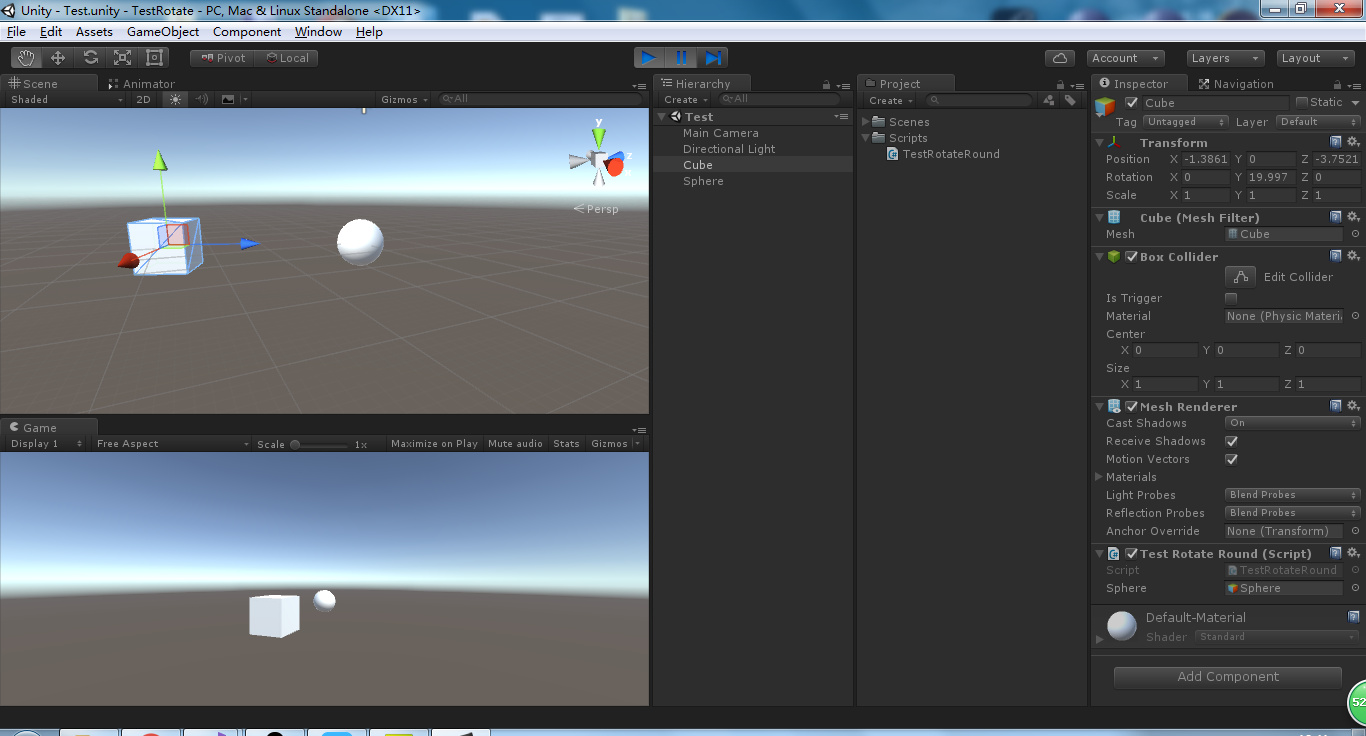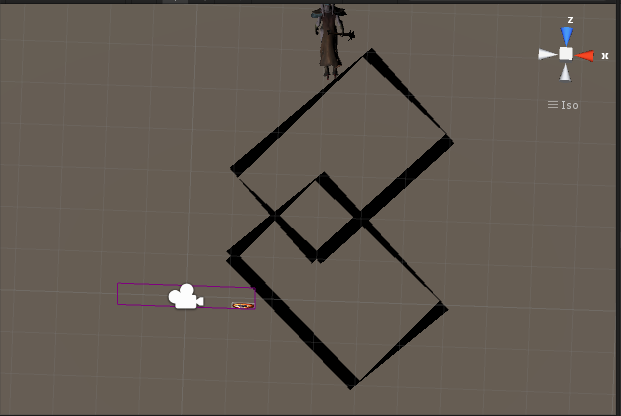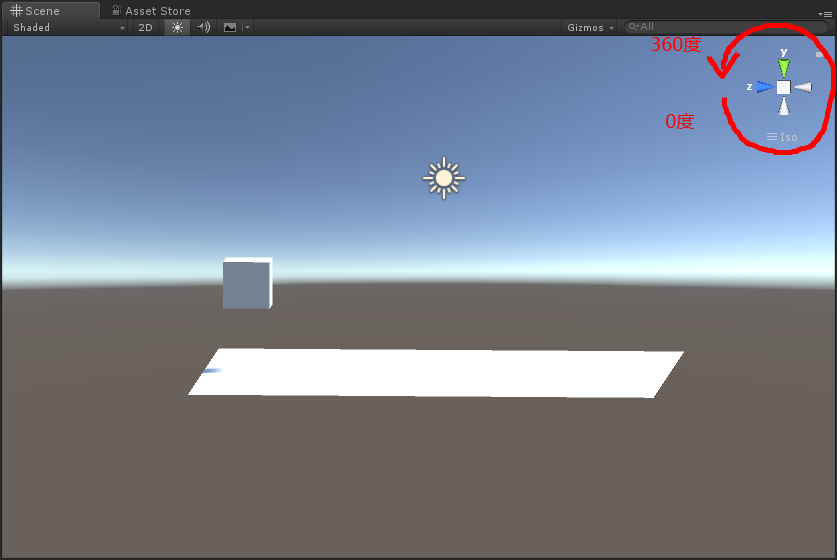• 先上一张效果图 using UnityEngine; using System.Collections; public class TestRotateRound : MonoBehaviour { public GameObject Sphere; private float curtTime = 0.0f; void Update() ... ...
先上一张效果图using UnityEngine;
using System.Collections;

public class TestRotateRound : MonoBehaviour
{
public GameObject Sphere;
private float curtTime = 0.0f;
void Update()
{
//使用C#封装好的代码RotateAround
gameObject.transform.RotateAround(Sphere.transform.position, Sphere.transform.up, 72 * Time.deltaTime);
//自己封装代码,功能和上面的相同
//RotateAround(Sphere.transform.position,Vector3.up, 72 * Time.deltaTime);
}

private void RotateAround(Vector3 center, Vector3 axis, float angle)
{
//绕axis轴旋转angle角度
Quaternion rotation = Quaternion.AngleAxis(angle, axis);
//旋转之前,以center为起点,transform.position当前物体位置为终点的向量.
Vector3 beforeVector = transform.position - center;
//四元数 * 向量(不能调换位置, 否则发生编译错误)
Vector3 afterVector = rotation * beforeVector;//旋转后的向量
//向量的终点 = 向量的起点 + 向量
transform.position = afterVector + center;

//看向Sphere,使Z轴指向Sphere
transform.LookAt(Sphere.transform.position);
}
}

展开全文unity 绕轴旋转
• 1，让个物体围绕一点旋转，有几种方法？分别是什么？ 答：在这个点处放个空物体B，则问题变为A着B旋转， 方法1：旋转函数transform.Rotate()来实现  方法2：B不动，A挂脚本实现transform的RotateAround...
1，让一个物体围绕某一点旋转，有几种方法？分别是什么？
答：在这个点处放一个空物体B，则问题变为A绕着B旋转，
方法1：B不动，A挂脚本实现transform的RotateAround(vector3 point, vector3 axis, float angle)函数
例如 A.RotateAround(B.position, Vector3.up,  30*Time.deltaTime);   //A绕着B的y轴进行旋转。
方法2：A不动，A作为B的子物体，B挂脚本实现自转，然后带着A转，类似于模拟围绕中心天体旋转。
例如：B.Rotate (Vector3.up, 30*Time.deltaTime, Space.Self); //B绕着自身的up方向自转，从而带着A一起转。
方法3：矩阵变换，研究中。
注：旋转的常用方法：
(1)绕坐标轴旋转

public void Rotate (Vector3 eulerAngles, Space relativeTo = Space.Self);或者

public void Rotate (float xAngle, float yAngle, float zAngleSpace, relativeTo = Space.Self);
其中relativeTo = Space.Self表示绕自身坐标系旋转，ralativeTo = Space.World表示绕世界坐标系旋转。
(2)饶某个向量旋转
public void Rotate(Vector3 axis, float angle, Space relativeTo);
其中axis为旋转轴方向，angle为旋转角度，relativeTo为参考坐标系，默认为Space.self。此方法的功能是使得GameObject对象在relativeTo坐标系中绕轴向量axis旋转angle度。
（3）绕轴点旋转

public void RotateAround(Vector3 point, Vector3 axis, float angel);

功能是使得GameObject对象绕着point点的axis方向旋转angle度。


展开全文unity
• 游戏中有需求，就是个矩形或者Cube一点旋转任意角度，现在给出下面算法。
游戏中有一需求，就是一个矩形或者Cube绕着某一点旋转任意角度，现在给出下面算法。
   public static Vector3 RotateRound(Vector3 position, Vector3 center, Vector3 axis, float angle)
{
Vector3 point = Quaternion.AngleAxis(angle, axis) * (position - center);
Vector3 resultVec3 = center + point;
return resultVec3;
}
测试用例
using UnityEngine;
using System.Collections;

public class RotateTest : MonoBehaviour
{
public LineRenderer line1;
public LineRenderer line2;
public float angle = 30f;

private Vector3 v0;
private Vector3 v1;
private Vector3 v2;
private Vector3 v3;
private Vector3 v4;
private Vector3 vCenter;
void Start()
{
v0 = new Vector3(3f,0f,1f);
v1 = new Vector3(1f, 0f, 3f);
v2 = new Vector3(4f, 0f, 6f);
v3 = new Vector3(6f, 0f, 4f);
vCenter = new Vector3(2f, 0f, 2f);
}

// Use this for initialization
void Update ()
{
line1.SetVertexCount(5);
line1.SetPosition(0,v0);
line1.SetPosition(1,v1);
line1.SetPosition(2,v2);
line1.SetPosition(3,v3);
line1.SetPosition(4,v0);

line2.SetVertexCount(5);
Vector3 v01 = MathUtils.RotateRound(v0, vCenter, Vector3.up, angle);
Vector3 v11 = MathUtils.RotateRound(v1, vCenter, Vector3.up, angle);
Vector3 v21 = MathUtils.RotateRound(v2, vCenter, Vector3.up, angle);
Vector3 v31 = MathUtils.RotateRound(v3, vCenter, Vector3.up, angle);
Vector3 v41 = MathUtils.RotateRound(v4, vCenter, Vector3.up, angle);
line2.SetPosition(0, v01);
line2.SetPosition(1, v11);
line2.SetPosition(2, v21);
line2.SetPosition(3, v31);
line2.SetPosition(4, v01);
}
}
效果图展开全文unity Rotate
• 在制作FPS相机时，遇到了需要限制角度的需求，视角只能查看到-60到60度的范围，而在Unity的Transform组件中，x逆时针旋转，Transform组件的localEulerAngle会在0~360范围内递增（如图） 关键在于其中的角度转换...在制作FPS相机时，遇到了需要限制角度的需求，视角只能查看到-60到60度的范围，而在Unity的Transform组件中，绕x轴逆时针旋转，Transform组件的localEulerAngle会在0~360范围内递增（如图）
关键在于其中的角度转换，直接上代码
        public static void RotateClampX(this Transform t, float degree, float min, float max)
{
degree = (t.localEulerAngles.x - degree);
if (degree > 180f)
{
degree -= 360f;
}

degree = Mathf.Clamp(degree, min, max);
t.localEulerAngles = t.localEulerAngles.SetX(degree);
}

参考了这位老兄的文章并稍微简化了一下
https://blog.csdn.net/qq_15386973/article/details/76255694


展开全文• Vector3 newVec = Quaternion.AngleAxis(angle,axis)*oriVec angle：旋转度数 axis:围绕哪个轴旋转 oriVec:初始向量
Vector3 newVec = Quaternion.AngleAxis(angle,axis)*oriVec
angle：旋转度数
axis:围绕哪个轴旋转
oriVec:初始向量


展开全文• 把以下代码绑定到要旋转的物体，具体要围绕x，y，z 哪个轴旋转就改相应的值即可。[csharp] view plain copyusing UnityEngine; using System.Collections; public class PickUp : MonoBehaviour { // ...
• 写在前面 阅读该文章之前，首先需要知道： ...本文的目的是探究游戏引擎Unity3D不同的旋转API的表现，我们都知道，在Unity3D中有多种刚体旋转的API：①有对localRotation进行赋值修改的方式达到旋转的目的；...
• 问题描述：昨天需要实现个功能是根据指令左转90度或者右转90度，当时脑汁可能是有浆糊吧，居然要用直接赋值rotation，这样一来就要牵扯到...、问题的解决：如何让物体自身轴旋转 直接上关键代码： playe...
• Unity 3D 中的旋转 Unity 3D 中 Rotation 在Unity中，旋转通常可以用个三维向量(x,y,z)表示。实际上这是欧拉角。三个分量分别是x、y和z旋转角度。 要对个GameObject进行旋转，可以直接通过...unity
• 那么下面就介绍任意单位轴旋转的两种方法。 假设要旋转的角度是a，围绕的轴是r。 方法： （1）构建新的基 寻找另外两条单位长度的坐标轴s、t，他们相互垂直，而且与r垂直。这样r、s、t
• 开始本人纠结于在VR中，怎么利用手柄来控制物体的旋转，物体位置不变。 相当于：地球仪。更通俗点来说，就是个棍子插到地球仪上，然后拿着棍子就可以控制地球仪转。手柄相当于那根棍子。 代码如下： ...Unity3D 绕轴旋转
• 1、四元数法 Vector3 newVec = Quaternion.AngleAxis(angle,axis)*oriVec; 或者 Vector3 newVec = Quaternion.Eular(0,angle,0)*oriVec; 2、二维向量运算公式： ...x1＝xcosθ+ysinθ, y1＝-xsinθ+ycosθunity
• 可以使用RotateAround，代码如下： transform.RotateAround (Sun.transform.position, Vector3.down, 5); ...第二个参数是指要围绕哪旋转，Vector3.down表示围绕y负向旋转（也可...
• 在Update函数中执行： if (turnAround) { playerCamera.RotateAround(transform.localPosition, transform.up, Time.deltaTime * rotateSpeed); degree += Time.deltaTime * rotateSpeed; ...
• 1，让个物体围绕一点旋转，有几种方法？分别是什么？ 答：在这个点处放个空物体B，则问题变为A着B旋转， 方法1：B不动，A挂脚本实现transform的RotateAround(vector3 point, vector3 axis, float angle)...
• unity3d中,用四元数来表示旋转,四元数英文名叫quaternion . 比如 transform.rotation 就是个四元数,其由四个部分组成  Quaternion = (xi + yj + zk + w ) = (x,y,z,w)    1. ...
• Unity中让游戏物体围绕自身的x、y、z进行旋转（亲测有效） void Update() { //当按着键盘的空格键时，物体就开始围绕自身的x进行旋转 if (Input.GetKey(KeyCode.Space)) { //如果是围绕自身的x...unity3d unity 游戏开发
• //功能实在很简单，放着吧using UnityEngine; using System.Collections; public class rotate : MonoBehaviour { Vector3 StartPosition;... //上帧鼠标的位置。 Vector3 offset; //在两帧c# unity
...